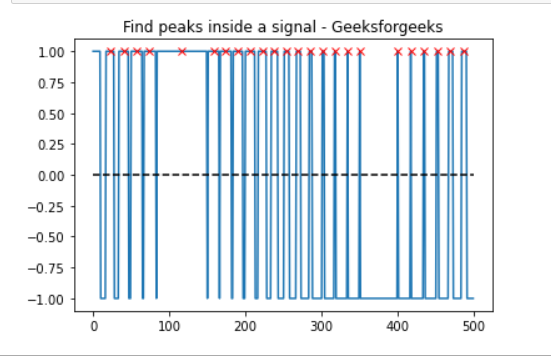Related Articles

# Find all peaks amplitude lies above 0 Using Scipy

• Last Updated : 26 Dec, 2020

Prerequisites: Mathplotlib, Scipy

In this article, we will see how to find all ‘x’ point above 0 with the help of find_peaks( ) function, that takes a 1-D array and finds all local maxima by a simple comparison of neighboring values.

Approach:

• Import required module.
• Find peaks inside a signal based on find_peaks() properties.
• Label the graph.
• Display Graph.

Step 1: Import all libraries.

## Python3

 `import` `matplotlib.pyplot as plt``import` `numpy as np``from` `scipy.signal ``import` `find_peaks``from` `scipy ``import` `signal`

Step 2: electrocardiogram(): The returned signal is a 5-minute-long electrocardiogram (ECG), a medical recording of the heart’s electrical activity, sampled at 360 Hz.

Syntax:

scipy.signal.find_peaks(x, height=None)

Parameter:

• x: A signal with peaks.
• height:  Required height of peaks. Either a number, None,

Return:

peaks: Indices of peaks in x that satisfy all given conditions.
peak_heights:  If the height is given, the height of each peak is x.

## Python3

 `import` `matplotlib.pyplot as plt``import` `numpy as np``from` `scipy.signal ``import` `find_peaks``from` `scipy ``import` `signal`` ` `t ``=` `np.linspace(``0``, ``1``, ``500``, endpoint``=``False``)``sig ``=` `np.sin(``2` `*` `np.pi ``*` `t)``x``=` `signal.square(``2` `*` `np.pi ``*` `30` `*` `t, duty``=``(sig ``+` `1``)``/``2``)``peak, _ ``=` `find_peaks(x, height``=``0``)`

Below is the full Implementation:

## Python3

 `import` `matplotlib.pyplot as plt``import` `numpy as np``from` `scipy.signal ``import` `find_peaks``from` `scipy ``import` `signal`` ` `t ``=` `np.linspace(``0``, ``1``, ``500``, endpoint``=``False``)``sig ``=` `np.sin(``2` `*` `np.pi ``*` `t)``x``=` `signal.square(``2` `*` `np.pi ``*` `30` `*` `t, duty``=``(sig ``+` `1``)``/``2``)``peak, _ ``=` `find_peaks(x, height``=``0``)`` ` `plt.plot(x)``plt.title(``"Find peaks inside a signal - Geeksforgeeks"``)``plt.plot(peak, x[peak], ``"x"``, color ``=` `'r'``)``plt.plot(np.zeros_like(x), ``"--"``, color``=``"black"``)``plt.show()`

Output:Attention geek! Strengthen your foundations with the Python Programming Foundation Course and learn the basics.

To begin with, your interview preparations Enhance your Data Structures concepts with the Python DS Course. And to begin with your Machine Learning Journey, join the Machine Learning – Basic Level Course

My Personal Notes arrow_drop_up